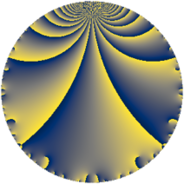# Properties

 Label 169.4.bLevel $169$ Weight $4$ Character orbit 169.b Rep. character $\chi_{169}(168,\cdot)$ Character field $\Q$ Dimension $34$ Newform subspaces $7$ Sturm bound $60$ Trace bound $4$

# Related objects

## Defining parameters

 Level: $$N$$ $$=$$ $$169 = 13^{2}$$ Weight: $$k$$ $$=$$ $$4$$ Character orbit: $$[\chi]$$ $$=$$ 169.b (of order $$2$$ and degree $$1$$) Character conductor: $$\operatorname{cond}(\chi)$$ $$=$$ $$13$$ Character field: $$\Q$$ Newform subspaces: $$7$$ Sturm bound: $$60$$ Trace bound: $$4$$ Distinguishing $$T_p$$: $$2$$

## Dimensions

The following table gives the dimensions of various subspaces of $$M_{4}(169, [\chi])$$.

Total New Old
Modular forms 52 44 8
Cusp forms 38 34 4
Eisenstein series 14 10 4

## Trace form

 $$34 q + 2 q^{3} - 118 q^{4} + 268 q^{9} + O(q^{10})$$ $$34 q + 2 q^{3} - 118 q^{4} + 268 q^{9} - 18 q^{10} + 10 q^{12} - 122 q^{14} + 466 q^{16} + 92 q^{17} + 424 q^{22} - 280 q^{23} - 356 q^{25} - 256 q^{27} + 350 q^{29} + 30 q^{30} + 304 q^{35} + 82 q^{36} + 202 q^{38} - 354 q^{40} + 52 q^{42} + 334 q^{43} - 552 q^{48} - 234 q^{49} - 216 q^{51} + 658 q^{53} - 912 q^{55} - 544 q^{56} - 950 q^{61} + 1190 q^{62} + 1292 q^{64} + 390 q^{66} - 1140 q^{68} + 700 q^{69} - 2272 q^{74} - 598 q^{75} - 1392 q^{77} + 1808 q^{79} - 2854 q^{81} - 1614 q^{82} - 1120 q^{87} + 2208 q^{88} + 3124 q^{90} + 1112 q^{92} - 1430 q^{94} + 248 q^{95} + O(q^{100})$$

## Decomposition of $$S_{4}^{\mathrm{new}}(169, [\chi])$$ into newform subspaces

Label Dim $A$ Field CM Traces $q$-expansion
$a_{2}$ $a_{3}$ $a_{5}$ $a_{7}$
169.4.b.a $2$ $9.971$ $$\Q(\sqrt{-1})$$ None $$0$$ $$-14$$ $$0$$ $$0$$ $$q+5iq^{2}-7q^{3}-17q^{4}+7iq^{5}-35iq^{6}+\cdots$$
169.4.b.b $2$ $9.971$ $$\Q(\sqrt{-3})$$ None $$0$$ $$-14$$ $$0$$ $$0$$ $$q-2\zeta_{6}q^{2}-7q^{3}-4q^{4}-8\zeta_{6}q^{5}+\cdots$$
169.4.b.c $2$ $9.971$ $$\Q(\sqrt{-1})$$ None $$0$$ $$4$$ $$0$$ $$0$$ $$q+4iq^{2}+2q^{3}-8q^{4}+17iq^{5}+8iq^{6}+\cdots$$
169.4.b.d $2$ $9.971$ $$\Q(\sqrt{-3})$$ None $$0$$ $$4$$ $$0$$ $$0$$ $$q-\zeta_{6}q^{2}+2q^{3}+5q^{4}-\zeta_{6}q^{5}-2\zeta_{6}q^{6}+\cdots$$
169.4.b.e $4$ $9.971$ $$\Q(i, \sqrt{17})$$ None $$0$$ $$10$$ $$0$$ $$0$$ $$q+(\beta _{1}+3\beta _{2})q^{2}+(1+3\beta _{3})q^{3}-5\beta _{3}q^{4}+\cdots$$
169.4.b.f $4$ $9.971$ $$\Q(i, \sqrt{17})$$ None $$0$$ $$10$$ $$0$$ $$0$$ $$q+\beta _{1}q^{2}+(1+3\beta _{3})q^{3}+(3+\beta _{3})q^{4}+\cdots$$
169.4.b.g $18$ $9.971$ $$\mathbb{Q}[x]/(x^{18} + \cdots)$$ None $$0$$ $$2$$ $$0$$ $$0$$ $$q+\beta _{9}q^{2}-\beta _{2}q^{3}+(-4+\beta _{1})q^{4}+(-\beta _{9}+\cdots)q^{5}+\cdots$$

## Decomposition of $$S_{4}^{\mathrm{old}}(169, [\chi])$$ into lower level spaces

$$S_{4}^{\mathrm{old}}(169, [\chi]) \cong$$ $$S_{4}^{\mathrm{new}}(13, [\chi])$$$$^{\oplus 2}$$